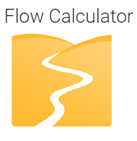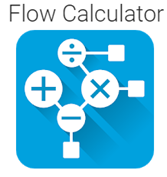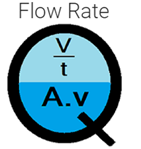# 7 – Best flow calculator apps for android and their features

1. Flow calculatorThis app can be used to calculate the open channel flow. This app can calculate the manning equation for different channel shapes. This app supports a rectangular, trapezoidal, triangular, and circular channel shape. This app also has certain theories related to manning equations and tables with values of all manning coefficients. By using this app we can calculate different parameters like velocity and discharge, channel slope from velocity and discharge, and water depth from discharge, and all these parameters can be calculated in a rectangular, circular, triangular, and trapezoidal channel.

2. Flow calculatorBy using this app we can calculate the volumetric flow. There will be a three-dimensional graphic representation and by clicking it we can calculate the depth or other flow parameters. This app also has an independent unit converter and this will help us to do the conversion of areas, dimensions, temperatures, etc. we can also choose between the metric and the imperial system of the unit.

3. Flow calculatorBy this app we can do multistep calculations, this app has an interactive interface everything can be dragged around and selected for further options and every number can be tagged with a text label. This app also supports many calculations and the calculations can be saved and loaded

4. Hydrowiz- Pipe flow hydraulic calculationsThis app can be very useful for flow calculations in pressurized pipes. This app can calculate the pressure loss, flow rate, pipe diameter, and pipe length. There are many options in this app and the user can select according to his need. It has preferred units of measurements in both SI/ Metric and US/ imperial units. This app also has a wide range of pipe materials and we can select the pipe roughness coefficient from it. The resistance coefficients can be selected from commonly used pipe fittings and valves. We can also select the fluid properties from a wide variety of options in the app.

5. Flow XpertBy using this app we can calculate the flow of liquid and gas, it can do the calculations for hydrocarbon liquids and gases and also for steam. It can be used as an oil and gas calculation library. This app is suitable for flow and fluid property calculations and can be used in flow and level measurement systems.

6. Flow rateThis app can be used to check the flow rate, it can be used as a volume flow rate calculator. This app has many equations and we can also do unit conversions by using this app.